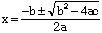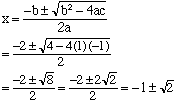Sender: Jason On Subject: Complex Number Question: Solve the following equations,expressing solutions in the form p + iq. sqr(x) + 2x - 1 = 0, sqr(x) + 2x - 3 = 0. Hi Jason, Your first example doesn't factor so to solve it you need to use the general quadratic which says that if a x2 = b x + c = 0 thenFor your example 1. a = 1, b = 2 and c = -1 soThis is in the form p + iq with q = 0. Cheers, Harley Go to Math Central